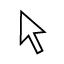# G.GCI.1 Proving Circles are Similar

Topic:
Circle
In the first semester we talked about transformations which produced congruent and similar figures.  See if you and your neighbor can remember which transformations produced which kinds of figures. Figures can be proven similar if one, or more, similarity transformations (reflections, translations, rotations, dilations) can be found that map one figure onto another. In our attempt to prove all circles are similar, a translation and a scale factor (from a dilation) will be found to map one circle onto another. We are going to prove circles are similar using transformations, but first let's practice on a pair of triangles.  I will give you the coordinates for the triangles in the applet below.  You will construct them using Geogebra, then experiment and decide which transformations (2) will map      ABC onto    JKL. You can select and move any figureyou construct.  Don't forget you can also zoom outor you can pan the coordinate plane across with the move graphics view button (top button on right)  in order to see all of your figures.       If there is a scale factor involved, be sure to record it and your transformations on your worksheet.

## Applet 1: Triangle ABC: A(-2, 7), B(-2, 3), C(1,3) Triangle DEF: D(3,7), E(3, -1), F(9, -1)

Now we are going to prove two circles are similar using the same method.  You can construct the following circles in the applet below.  Be sure to record the transformations which you used to map the first circle onto the second onto your worksheet.

## Applet 3

For this example, I will give you the coordinates for the center of each circle, and an endpoint for each  radius.  It is up to you to find the lengths of the radii, and decide on the transformations needed to map one circle onto the other.  Circle 1:  center (0,0) point on circle (2,3) Circle 2:  center (10,2) point on circle (18, 14) There are many ways to obtain the length of the radius.

## Applet 4:

Given the centers and one point on the circle, find the transformations which will map the first circle onto the other.